## Animals

The Animals program has been used in many programming tutorials because it's fun and simple to implement, and demonstrates how a computer can interactively build a database with input from the user. It can be written quite elegantly in Lisp by taking advantage of the language's powerful list handling.

Complete listing

### Description

The Animals game tries to guess the animal you are thinking of. To play the game you simply type:

`CL-USER > (play-animals)`

The program then asks a series of questions, such as: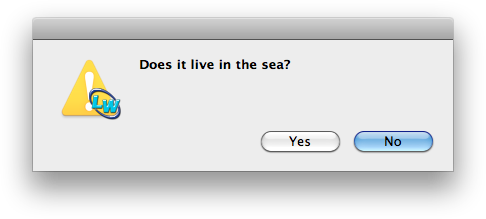to which you answer Yes or No as appropriate. Eventually it makes a guess at the animal:If it was correct the game ends. However, if you clicked No the program prompts: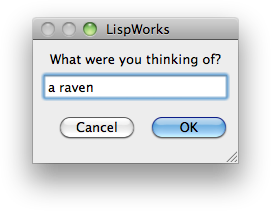You are then prompted to add a question to the tree: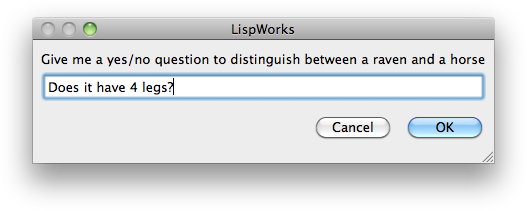Finally the program needs to know the correct answer for the new animal: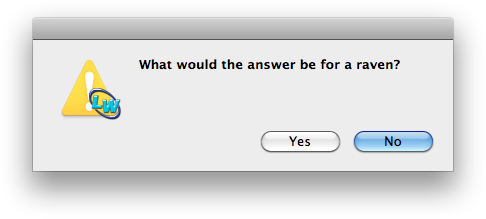As a result of this interaction the new animal has been added to the database, and the next time you play the program will know about the new animal.

### Designing the program

We will represent the database as a tree of questions and animals. The program starts with one question and two animals: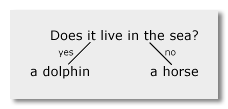We'll represent the tree as a Lisp list, of the form:

`(question yes-answer no-answer)`

So for the tree shown above the list will be:

`("Does it live in the sea?" "a dolphin" "a horse")`

We will store the database tree in a variable called *tree*, so we'll start by defining:

`(defparameter animals-tree '("Does it live in the sea?" "a dolphin" "a horse"))`

After it has been trained with two more animals the tree might look like this: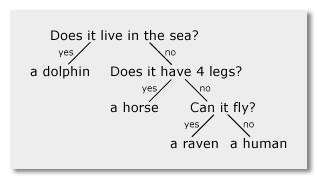Here's the Lisp representation, indented to make the structure clearer:

```("Does it live in the sea?"
"a dolphin"
("Does it have 4 legs?"
"a horse"
("Can it fly?"
"a raven"
"a human")))```

We're going to build the animals program out of four separate functions: learn-animal, make-guess, ask-question, and animals. Although we could have combined some of these functions, the advantage of breaking it down into small, separate building-blocks is that we can test each function independently.

### Learning a new animal

First let's define the procedure to learn a new animal:

```(defun learn-animal (animal new-animal)
(let ((question
(capi:prompt-for-string
(format nil "Give me a yes/no question to distinguish between ~a and ~a"
new-animal animal))))
(if (capi:prompt-for-confirmation
(format nil "What would the answer be for ~a?" new-animal))
(list question animal new-animal))
(list question new-animal animal)))```

This takes two parameters: an existing animal, and a new animal. It prompts for a question, and the correct answer for the new animal, and returns a list of the question and answers in the correct order. For example:

`(learn-animal "a cat" "a dog")`

will prompt:

Give me a yes/no question to distinguish between a dog and a cat

and

What would the answer be for a dog?

and return the appropriate list; for example

`("Does it bark?" "a dog" "a cat")`

### Making a guess

The next procedure, make-guess, is called when we've reached an animal on the tree. It takes the animal as its parameter, and guesses that as the answer. If it's correct it simply returns the animal. If it's incorrect, it calls learn-animal to create a list containing a new question and the two answers.

```(defun make-guess (animal)
(if (capi:prompt-for-confirmation (format nil "Is it ~a?" animal))
(let ((message (capi:display-message "Ho ho!")))
animal)
(learn-animal animal (capi:prompt-for-string "What were you thinking of?"))))
```

The next procedure, ask-question, is called when we're at a question on the tree. It prompts with the question, and depending on whether the answer is yes or no it calls animals on the left branch or right branch of the tree respectively.

```(defun ask-question (tree)
(if (capi:prompt-for-confirmation (first tree))
(list (first tree) (animals (second tree)) (third tree))
(list (first tree) (second tree) (animals (third tree)))))```

It returns the complete tree, modified to add a new animal if one was added.

### The main procedure - animals

Finally here's the main procedure, animals. It is called with the current position on the tree as the parameter, and simply calls ask-question if the parameter is a list, indicating that more questions need to be asked, or make-guess if it's an animal, indicating that we're at the bottom of a branch.

```(defun animals (tree)
(if (listp tree)
(make-guess tree)))```

To save the modified tree we need to save it back as *tree*. Here's a routine play-animals that does that for us:

```(defun play-animals ()
(setf animals-tree (animals animals-tree)))```

### Saving the tree

Note that the value of *tree* should be saved to disk if you want to preserve the results of training your database with new animals. Here's a modified version of play-animals that does that (rather crudely), saving the data in a file called tree at the top level of your hard disk. Note that this uses functions that we don't explain in these tutorials so you'll have to take them on trust:

```(defun play-animals ()
(when (probe-file "tree")
(with-open-file (stream "tree" :direction :input)